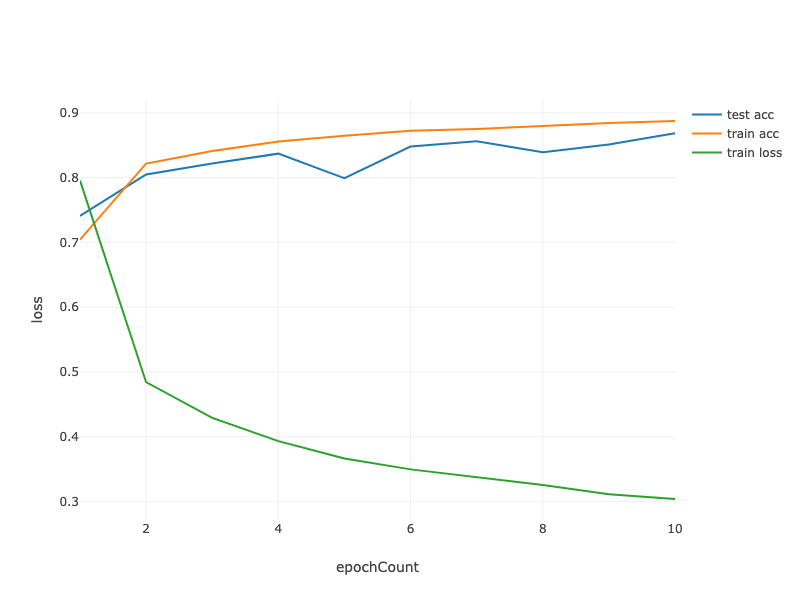# 4.2. Implementation of Multilayer Perceptron from Scratch¶

Now that we have characterized multilayer perceptrons (MLPs) mathematically, let us try to implement one ourselves.

%mavenRepo snapshots https://oss.sonatype.org/content/repositories/snapshots/

%maven ai.djl:api:0.7.0-SNAPSHOT
%maven ai.djl:basicdataset:0.7.0-SNAPSHOT
%maven org.slf4j:slf4j-api:1.7.26
%maven org.slf4j:slf4j-simple:1.7.26
%maven ai.djl.mxnet:mxnet-engine:0.7.0-SNAPSHOT
%maven ai.djl.mxnet:mxnet-native-auto:1.7.0-b

%%loadFromPOM
<dependency>
<groupId>tech.tablesaw</groupId>
<artifactId>tablesaw-jsplot</artifactId>
<version>0.30.4</version>
</dependency>

%load ../utils/plot-utils.ipynb

import ai.djl.Device;
import ai.djl.engine.Engine;
import ai.djl.ndarray.NDArray;
import ai.djl.ndarray.NDList;
import ai.djl.ndarray.NDManager;
import ai.djl.ndarray.types.DataType;
import ai.djl.ndarray.types.Shape;
import ai.djl.training.dataset.Batch;
import ai.djl.training.dataset.Dataset;
import ai.djl.training.loss.Loss;
import ai.djl.training.optimizer.Optimizer;
import ai.djl.training.tracker.Tracker;
import ai.djl.basicdataset.FashionMnist;
import ai.djl.training.util.ProgressBar;

import tech.tablesaw.api.*;
import tech.tablesaw.plotly.api.*;
import tech.tablesaw.plotly.components.*;
import tech.tablesaw.plotly.Plot;
import tech.tablesaw.plotly.components.Figure;
import org.apache.commons.lang3.ArrayUtils;


To compare against our previous results achieved with (linear) softmax regression (Section 3.6), we will continue work with the Fashion-MNIST image classification dataset (Section 3.5).

int batchSize = 256;

FashionMnist trainIter = FashionMnist.builder()
.optUsage(Dataset.Usage.TRAIN)
.setSampling(batchSize, true)
.build();

FashionMnist testIter = FashionMnist.builder()
.optUsage(Dataset.Usage.TEST)
.setSampling(batchSize, true)
.build();

trainIter.prepare();
testIter.prepare();


## 4.2.1. Initializing Model Parameters¶

Recall that Fashion-MNIST contains $$10$$ classes, and that each image consists of a $$28 \times 28 = 784$$ grid of (black and white) pixel values. Again, we will disregard the spatial structure among the pixels (for now), so we can think of this as simply a classification dataset with $$784$$ input features and $$10$$ classes. To begin, we will implement an MLP with one hidden layer and $$256$$ hidden units. Note that we can regard both of these quantities as hyperparameters and ought in general to set them based on performance on validation data. Typically, we choose layer widths in powers of $$2$$, which tend to be computationally efficient because of how memory is alotted and addressed in hardware.

Again, we will represent our parameters with several NDArrays. Note that for every layer, we must keep track of one weight matrix and one bias vector. As always, we call attachGradient() to allocate memory for the gradients (of the loss) with respect to these parameters.

int numInputs = 784;
int numOutputs = 10;
int numHiddens = 256;

NDManager manager = NDManager.newBaseManager();

NDArray W1 = manager.randomNormal(
0, 0.01f, new Shape(numInputs, numHiddens), DataType.FLOAT32, Device.defaultDevice());
NDArray b1 = manager.zeros(new Shape(numHiddens));
NDArray W2 = manager.randomNormal(
0, 0.01f, new Shape(numHiddens, numOutputs), DataType.FLOAT32, Device.defaultDevice());
NDArray b2 = manager.zeros(new Shape(numOutputs));

NDList params = new NDList(W1, b1, W2, b2);

for (NDArray param : params) {
}


## 4.2.2. Activation Function¶

To make sure we know how everything works, we will implement the ReLU activation ourselves using the maximum function rather than invoking Activation.relu directly.

public NDArray relu(NDArray X){
return X.maximum(0f);
}


## 4.2.3. The model¶

Because we are disregarding spatial structure, we reshape each 2D image into a flat vector of length numInputs. Finally, we implement our model with just a few lines of code.

public NDArray net(NDArray X) {

X = X.reshape(new Shape(-1, numInputs));
}


## 4.2.4. The Loss Function¶

To ensure numerical stability, and because we already implemented the softmax function from scratch (Section 3.6), we leverage Gluon’s integrated function for calculating the softmax and cross-entropy loss. Recall our earlier discussion of these intricacies (Section 4.1). We encourage the interested reader to examine the source code for Loss.SoftmaxCrossEntropyLoss to deepen their knowledge of implementation details.

Loss loss = Loss.softmaxCrossEntropyLoss();


## 4.2.5. Training¶

Fortunately, the training loop for MLPs is exactly the same as for softmax regression.

We run the training like how we did in Chapter 3, (see Section 3.6), setting the number of epochs to $$10$$ and the learning rate to $$0.5$$.

int numEpochs = 10;
float lr = 0.5f;

double[] trainLoss;
double[] testAccuracy;
double[] epochCount;
double[] trainAccuracy;

trainLoss = new double[numEpochs];
trainAccuracy = new double[numEpochs];
testAccuracy = new double[numEpochs];
epochCount = new double[numEpochs];

float epochLoss = 0f;
float accuracyVal = 0f;

for (int epoch = 1; epoch <= numEpochs; epoch++) {

System.out.print("Running epoch " + epoch + "...... ");
// Iterate over dataset
for (Batch batch : trainIter.getData(manager)) {

NDArray yHat = net(X); // net function call

NDArray lossValue = loss.evaluate(new NDList(y), new NDList(yHat));
NDArray l = lossValue.mul(batchSize);

accuracyVal += Training.accuracy(yHat, y);
epochLoss += l.sum().getFloat();

}

batch.close();
Training.sgd(params, lr, batchSize); // updater
}

trainLoss[epoch-1] = epochLoss/trainIter.size();
trainAccuracy[epoch-1] = accuracyVal/trainIter.size();

epochLoss = 0f;
accuracyVal = 0f;
// testing now
for (Batch batch : testIter.getData(manager)) {

NDArray yHat = net(X); // net function call
accuracyVal += Training.accuracy(yHat, y);
}

testAccuracy[epoch-1] = accuracyVal/testIter.size();
epochCount[epoch-1] = epoch;
accuracyVal = 0f;
System.out.println("Finished epoch " + epoch);
}

System.out.println("Finished training!");

Running epoch 1...... Finished epoch 1
Running epoch 2...... Finished epoch 2
Running epoch 3...... Finished epoch 3
Running epoch 4...... Finished epoch 4
Running epoch 5...... Finished epoch 5
Running epoch 6...... Finished epoch 6
Running epoch 7...... Finished epoch 7
Running epoch 8...... Finished epoch 8
Running epoch 9...... Finished epoch 9
Running epoch 10...... Finished epoch 10
Finished training!

String[] lossLabel = new String[trainLoss.length + testAccuracy.length + trainAccuracy.length];

Arrays.fill(lossLabel, 0, trainLoss.length, "train loss");
Arrays.fill(lossLabel, trainAccuracy.length, trainLoss.length + trainAccuracy.length, "train acc");
Arrays.fill(lossLabel, trainLoss.length + trainAccuracy.length,
trainLoss.length + testAccuracy.length + trainAccuracy.length, "test acc");

StringColumn.create("lossLabel", lossLabel)
);

render(LinePlot.create("", data, "epochCount", "loss", "lossLabel"),"text/html");Fig. 4.2.1 lineplot

## 4.2.6. Summary¶

We saw that implementing a simple MLP is easy, even when done manually. That said, with a large number of layers, this can still get messy (e.g., naming and keeping track of our model’s parameters, etc).

## 4.2.7. Exercises¶

1. Change the value of the hyperparameter numHiddens and see how this hyperparameter influences your results. Determine the best value of this hyperparameter, keeping all others constant.

2. Try adding an additional hidden layer to see how it affects the results.

3. How does changing the learning rate alter your results? Fixing the model architecture and other hyperparameters (including number of epochs), what learning rate gives you the best results?

4. What is the best result you can get by optimizing over all the parameters (learning rate, iterations, number of hidden layers, number of hidden units per layer) jointly?

5. Describe why it is much more challenging to deal with multiple hyperparameters.

6. What is the smartest strategy you can think of for structuring a search over multiple hyperparameters?